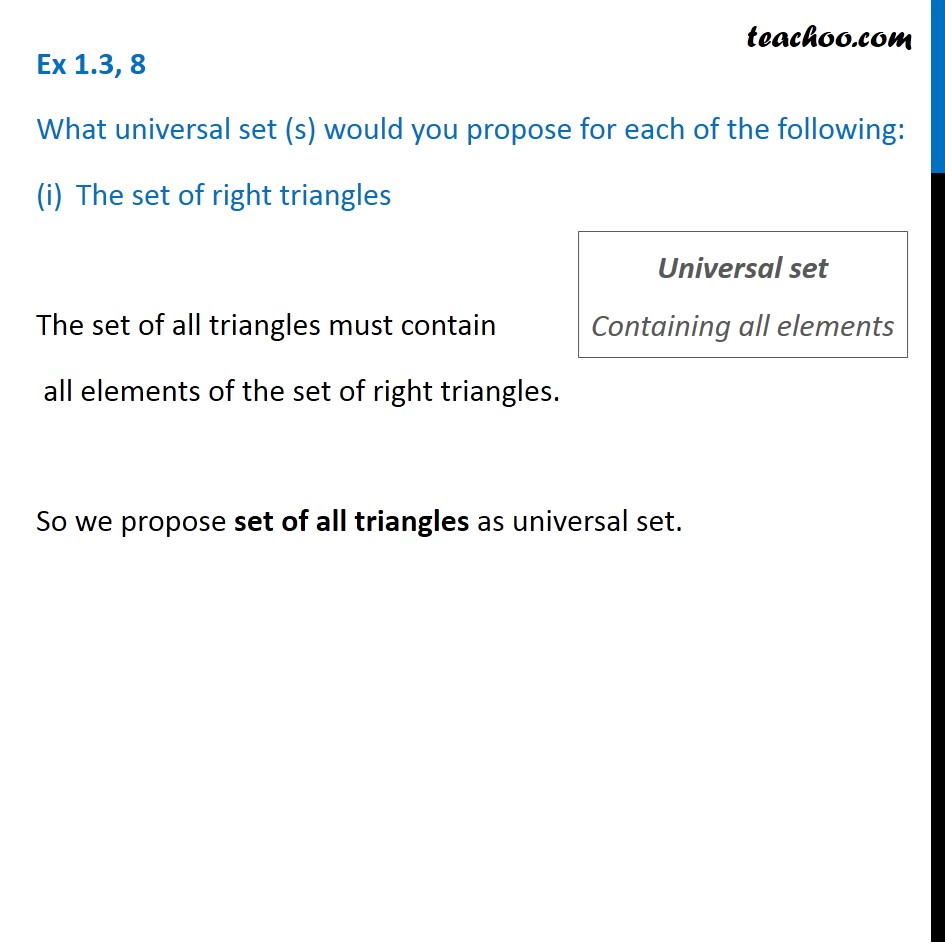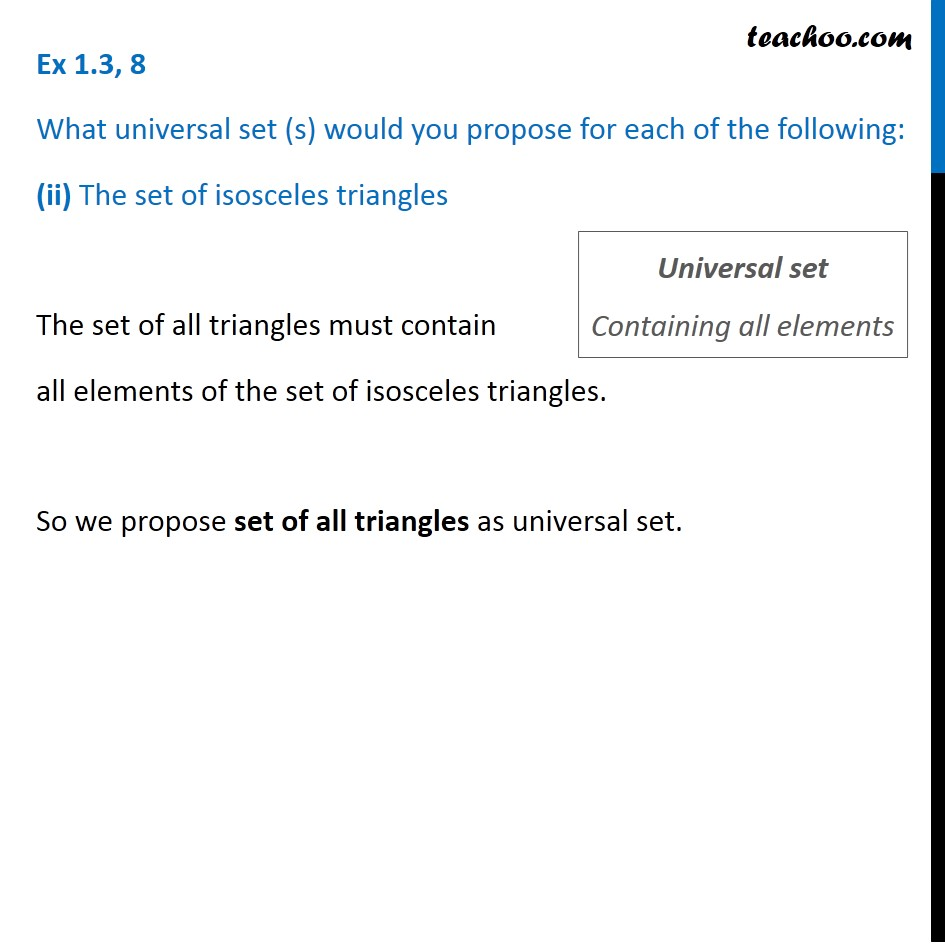Universal Set

Chapter 1 Class 11 Sets
Concept wiseIntroducing your new favourite teacher - Teachoo Black, at only ₹83 per month

### Transcript

Ex 1.3, 8 What universal set (s) would you propose for each of the following: The set of right triangles The set of all triangles must contain all elements of the set of right triangles. So we propose set of all triangles as universal set. Universal set Containing all elements Ex 1.3, 8 What universal set (s) would you propose for each of the following: (ii) The set of isosceles triangles The set of all triangles must contain all elements of the set of isosceles triangles. So we propose set of all triangles as universal set. Universal set Containing all elements